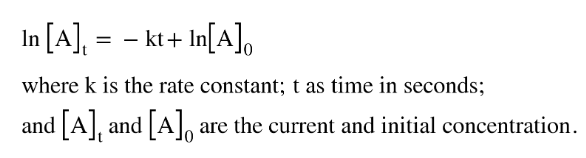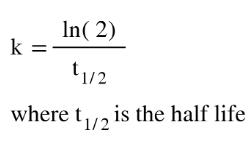# Problem: A first-order reaction is 75.0% complete in 320. s.a. What are the first and second half-lives for this reaction?

###### FREE Expert Solution
• We will be using the first order equation where:• Calculate k for this problem by plugging in the [A]t , [A]0 and t
• The k calculated can be used to get the first half life.• Second half life will be double of the first half life
92% (129 ratings)###### Problem Details

A first-order reaction is 75.0% complete in 320. s.

a. What are the first and second half-lives for this reaction?